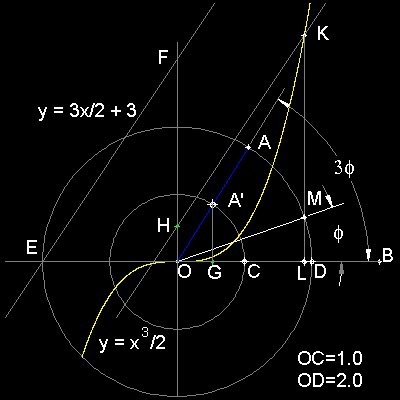Special Curves
Go to   Fun_Math Content Table   Trisecting an Angle   Special Curves

### Trisection using Special Curves

#### The Cubic Parabola

The idea of using Parabola can be easily extended to "Cubic parabola". The idea is shown in the figure shown below.##### Formulation of Cubic Parabola
Expanding the idea of the previous method using Parabola, Cubic Parabola can also be used for angle trisection.

Dividing all 3 terms of the Trisection Equation by 2, the result will be

(1/2)x3 = (3/2)x + a

This suggests that the roots of the trisection equation are the x-values of the intersection of cubic parabola and a line cutting y-axis at y = a with its slope = 3/2.
******** cubic_parabola_tri_desc.dwg ********

You can see the process in animation.animation

To create this drawing and animation:
Then from command line, type cubic_trisection

Example: AOB = 60 degrees case1. Input 2<60. to specify point A
2. Line AO(blue) will cut inner circle at A'.
3. Drop a line from A' perpendiculr to x-axs, and locate point G(green).
4. Find a point H on y-axis such that OG = OH
5. Draw a line through H parallel to a line EF
( y = 3x/2 + 3).
6. This line intersects cubic parabola at point K.
7. Drop a line from point K perpendicular to x-axis.
8. This line intersects the outer circle at point M.
9. angle MOB trisects angle AOB.

Question: How about the other two intersecting points ?
** cubic_parabola_trisection_60_deg.dwg **

#### References

Go to   Fun_Math Content Table   Trisecting an Angle   Special Curves

All questions/suggestions should be sent to Takaya Iwamoto

Last Updated Nov 22, 2006